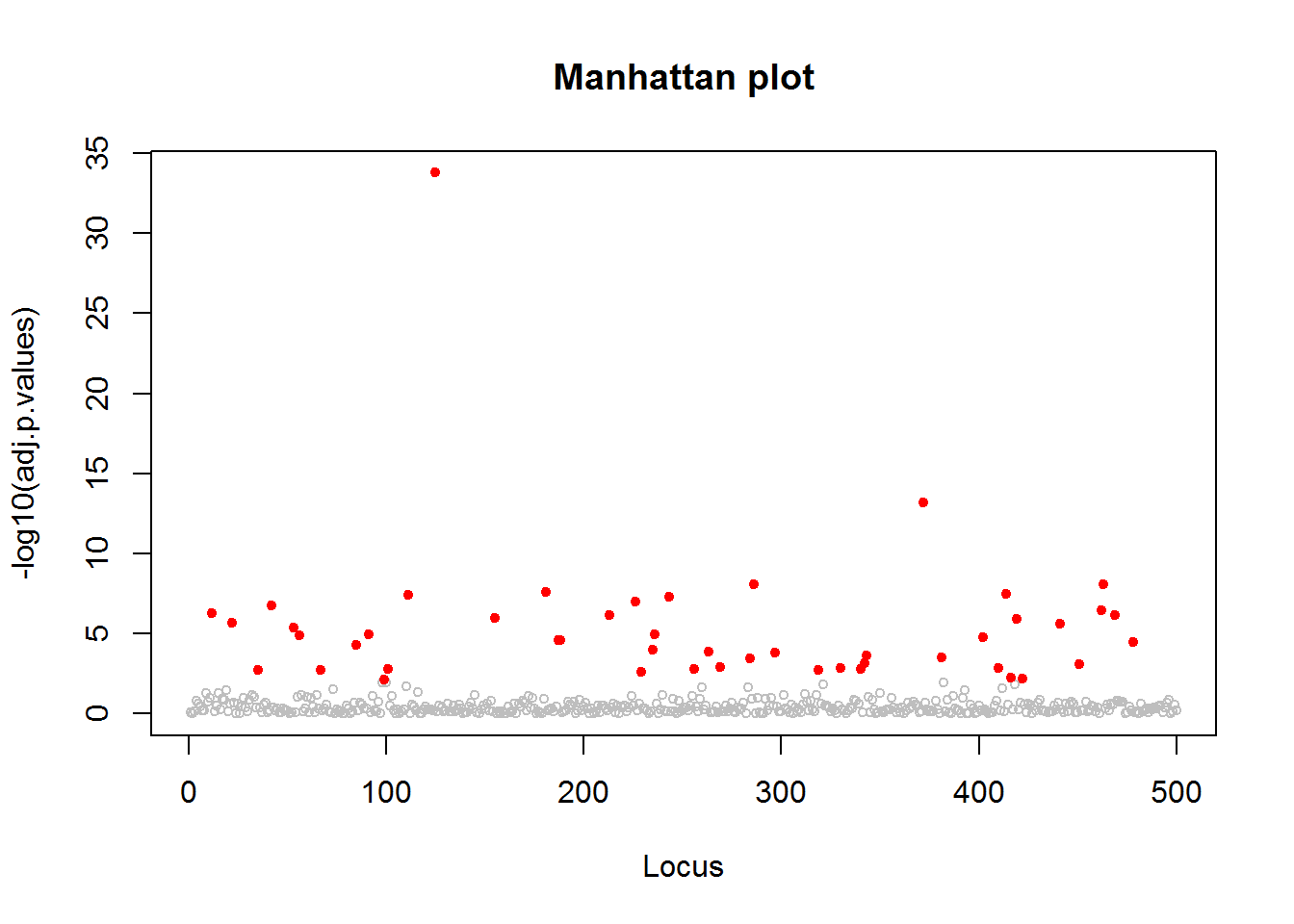This short tutorial illustrates how to analyze population genetic structure, and how to perform genome scans for selection using the function snmf from the R computer package LEA (Frichot et al. 2014, Frichot et al. 2015). This tutorial also provides guidelines for controlling the false discovery rate in genome scans for selection.

LEA can handle several classical formats for genotypic matrices. More specifically, the package uses the lfmm and geno formats, and provides functions to convert data sets in ped, vcf, or ancestrymap format.

The example data set used in the demo is available here: genotype1.lfmm.

A LEA session starts with the following R command

library(LEA)
## Warning: package 'LEA' was built under R version 3.2.0

## Analysis of population structure

Using the genotypic matrix, the first step is to evaluate population genetic structure. The snmf function provides outputs similar to those of the computer program STRUCTURE. We start with converting the genotype matrix from the “lfmm” to the “geno” format as follows

genotype = lfmm2geno("genotype1.lfmm")
##
##  - number of detected individuals:   200
##  - number of detected loci:      500

Then we create an “snmf” object (or project) using the snmf function. Here we run the program for $$K= 1-6$$ ancestral populations.

obj.snmf = snmf(genotype, K = 1:6, entropy = T, ploidy = 2, project = "new")
## The project is saved into :
##  genotype1.snmfProject
##
## To load the project, use:
##
## To remove the project, use:
##  remove.snmfProject("genotype1.snmfProject")


Since the program was called with the entropy option, we can plot the values of the cross-entropy criterion for each $$K$$. The value for which the function plateaus or increases is our estimate of $$K$$.

plot(obj.snmf)
## Warning in plot(s\$crossEntropy[1, ], ylab = "Minimal Cross-Entropy", xlab
## = "Number of ancestral populations", : bytecode version mismatch; using
## eval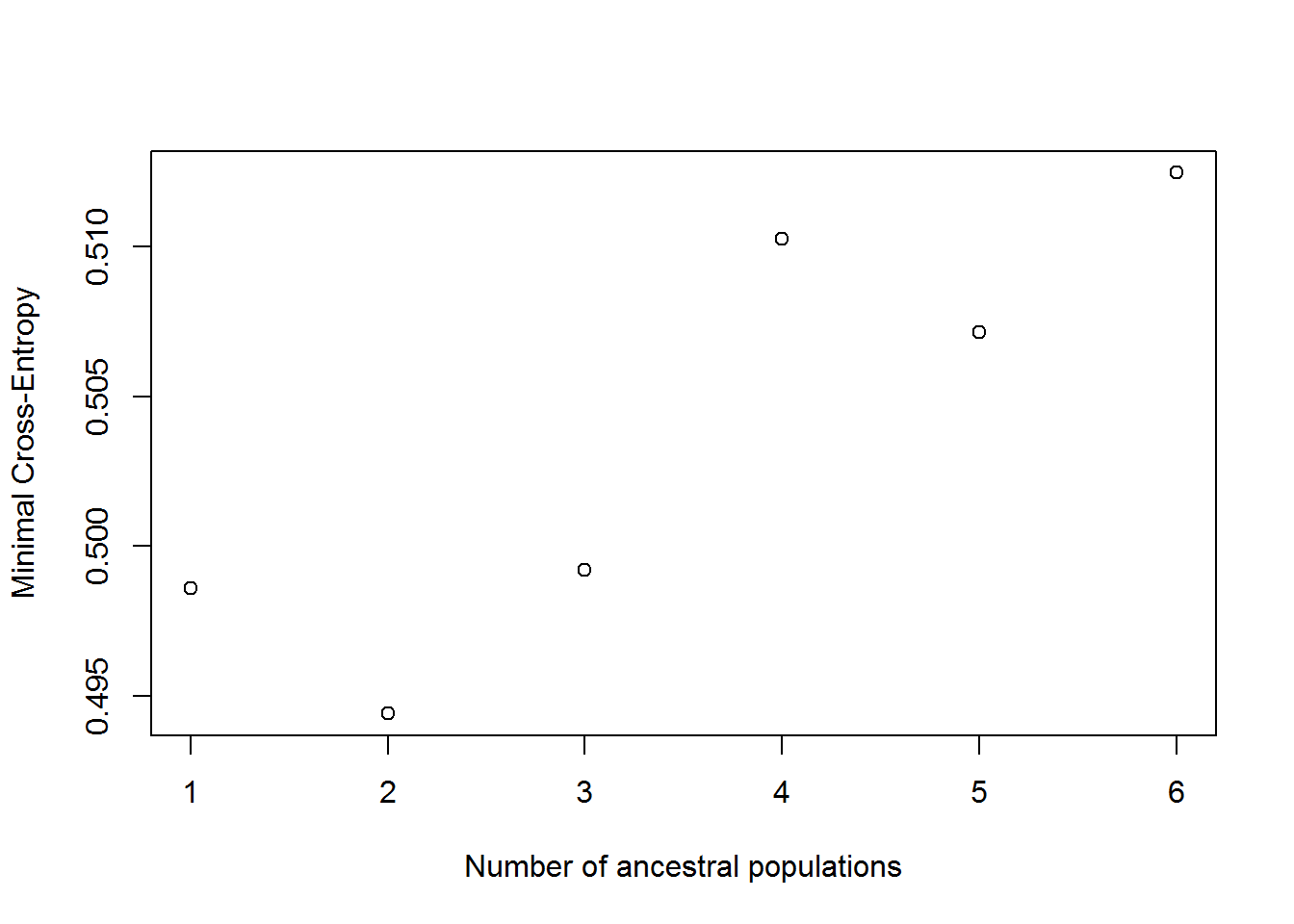We observe that the minumum value is obtained for $$K = 2$$. Note that we can visualize the barplot of ancestry coeffcients as follows.

barplot(t(Q(obj.snmf, K = 2)), col = 2:3, ylab = "Ancestry coefficients", xlab = "Sampled individuals")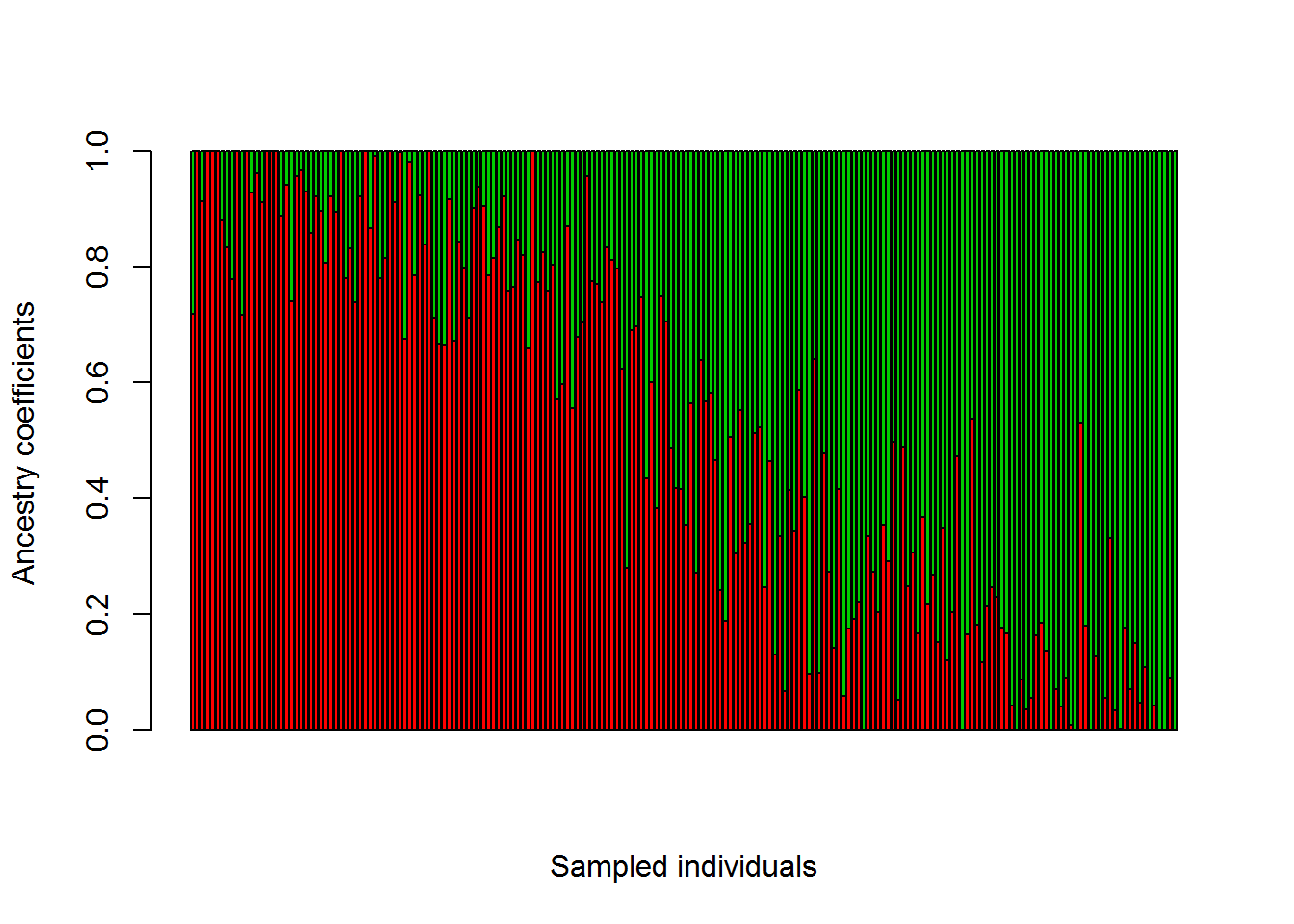## Genome scan for selection using an ancestral allele frequency differentiation test

The next step of analysis is to run a genome scan for selection based on the $$F_{\text ST}$$ statistic. Here, the $$F_{\text ST}$$ statistic is computed from the ancestral allele frequencies estimated by snmf.We define the function fst function as follows.

fst = function(project,run = 1, K, ploidy = 2){
library(LEA)
l = dim(G(project, K = K, run = run))
q = apply(Q(project, K = K, run = run), MARGIN = 2, mean)
if (ploidy == 2) {
G1.t = G(project, K = K, run = run)[seq(2,l,by = 3),]
G2.t = G(project, K = K, run = run)[seq(3,l,by = 3),]
freq = G1.t/2 + G2.t}
else {
freq = G(project, K = K, run = run)[seq(2,l,by = 2),]}
H.s = apply(freq*(1-freq), MARGIN = 1, FUN = function(x) sum(q*x))
P.t = apply(freq, MARGIN = 1, FUN = function(x) sum(q*x))
H.t = P.t*(1-P.t)
return(1-H.s/H.t)
}

Then we compute the $$F_{\text ST}$$ statistics as follows

fst.values = fst(obj.snmf, K=2)

Next, we convert the $$F_{\text ST}$$ values into absolute values of $$z$$-scores.

#convert fst values into z-scores (absolute values)
n = dim(Q(obj.snmf, K = 2))
fst.values[fst.values<0] = 0.000001
K = 2
z.scores = sqrt(fst.values*(n-K)/(1-fst.values))

### Computing a correct set of $$p$$-values

The estimated $$z$$-scores must be recalibrated before applying a test for neutrality at each locus. Due to the existence of population structure, the null-hypothesis may be misspecified, and this step is often necessary.

We suggest using an “empirical-null hypothesis” approach, starting with computing a genomic inflation factor, $$\lambda$$, and then dividing the scores by $$\lambda$$

.
#Compute the GIF
K=2
lambda = median(z.scores^2)/qchisq(1/2, df = K-1)
lambda
##  1.784576
# compute adjusted p-values from the combined z-scores
adj.p.values = pchisq(z.scores^2/lambda, df = K-1, lower = FALSE)

Then, we can check the histogram of $$p$$-values. Ideally, this histogram should have a flat shape with a peak close to zero. Having this shape indicates that the null-hypothesis is correct, i.e., $$p$$-values are sampled from a uniform distribution under the null-hypothesis.

#histogram of p-values
hist(adj.p.values, col = "red")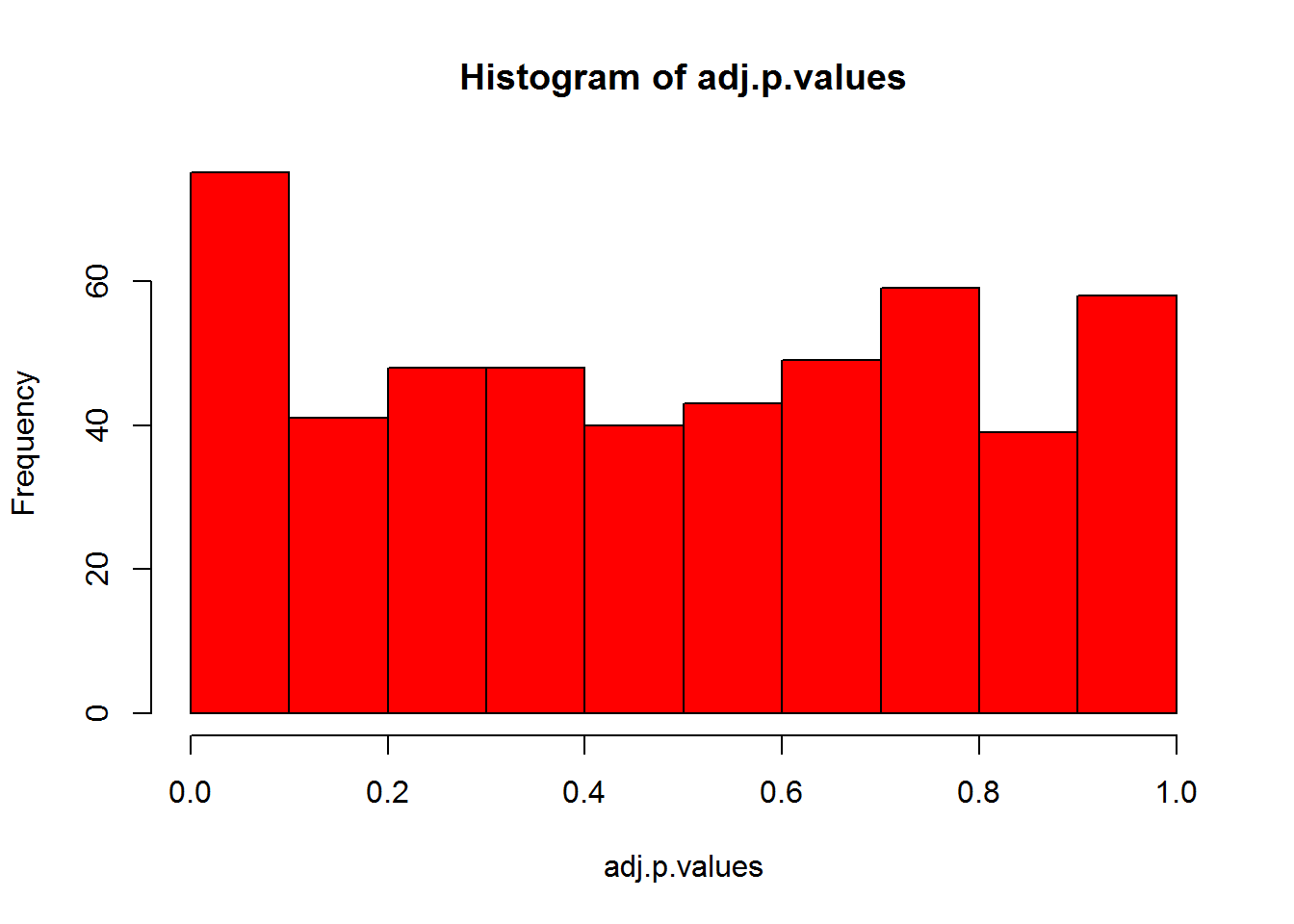The genomic inflation factor is acknowledged to be overly conservative. So, it might be better to calibrate the $$p$$-values using other methods (eg, using the R package “fdrtool") or following a trial-and-error approach. Here we try $$\lambda = 1.6$$.

# compute adjusted p-values from the combined z-scores
adj.p.values = pchisq(z.scores^2/1.6, df = 1, lower = FALSE)

#histogram of p-values
hist(adj.p.values, col = "green")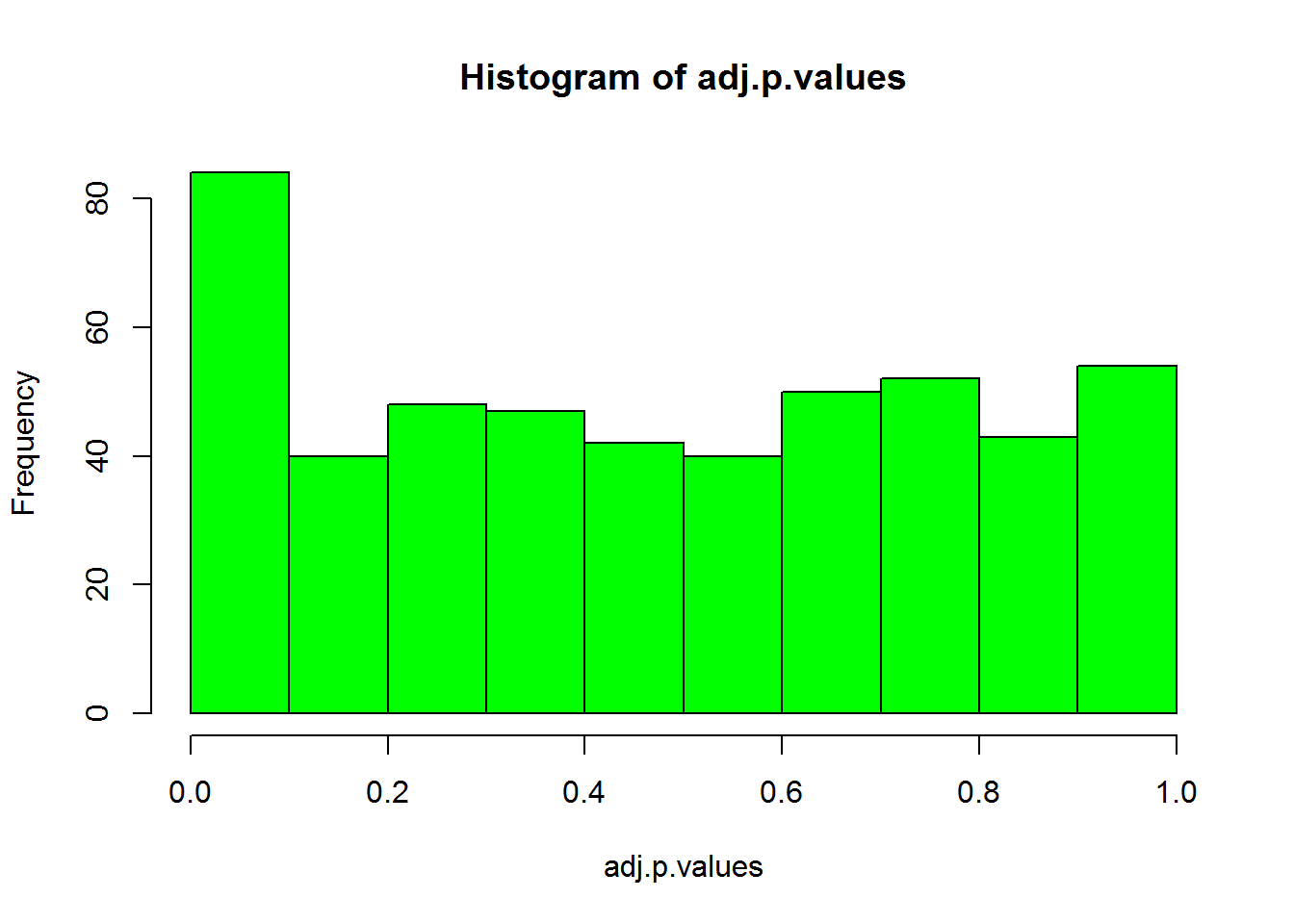The plot looks slightly better.

### Control of false discoveries

To correct for multiple testing, we apply the Benjamini-Hochberg algorithm. This can be done as follows.

## FDR control: Benjamini-Hochberg at level q
L = 500
q = 0.1
w = which(sort(adj.p.values) < q * (1:L)/L)
candidates = order(adj.p.values)[w]

Now, our list of candidate loci is recorded in the R object “candidates”.

plot(-log10(adj.p.values), main="Manhattan plot", xlab = "Locus", cex = .7, col = "grey")

points(candidates, -log10(adj.p.values)[candidates], pch = 19, cex = .7, col = "red")